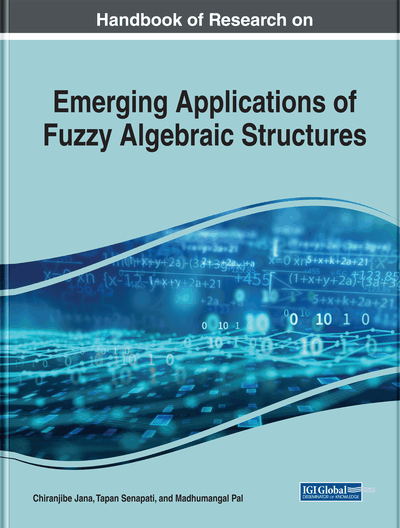Fuzzy Bi-Interior Ideals of Semirings

Venkateswarlu Bollineni (GITAM University, Bengaluru Rural, India), Sri Lakshmi T. (GITAM University, Visakhapatnam, India) and Adi Narayana Y. (NRI IT, Vijayawada, India)
DOI: 10.4018/978-1-7998-0190-0.ch009
Available
\$37.50
No Current Special Offers

Abstract

In this chapter, the authors introduce the notion of a fuzzy bi-interior ideal of semiring and they characterize the regular semiring in terms of fuzzy bi-interior ideal of semiring. And also they proved Let I be a non-empty subset of a semiring M and χ_I be the characteristic function of I. Then I is a bi interior ideal of semiring if and only if χ_I is a fuzzy bi-interior ideal of semiring M.
Chapter Preview
Top

1 Introduction

Semiring is an algebraic structure which is a common generalization of rings and distributive lattices, was first introduced by Vandiver (1934) but non-trivial examples of semirings had appeared in the studies on the theory of commutative ideals of rings by Dedekind in 19th century. Semiring is a universal algebra with two binary operations called addition and multiplication, where one of them distributive over the other. Bounded distributive lattices are commutative semirings which are both additively idempotent and multiplicatively idempotent. A natural example of semiring is the set of all natural numbers under usual addition and multiplication of numbers. In particular, if I is the unit interval on the real line, then (I, max, min) is a semiring in which 0 is the additive identity and 1 is the multiplicative identity. The theory of rings and the theory of semigroups have considerable impact on the development of the theory of semirings. In structure, semirings lie between semigroups and rings. Additive and multiplicative structures of a semiring play an important role in determining the structure of a semiring. Semiring as the basic algebraic structure was used in the areas of theoretical computer science as well as in the solutions of graph theory, optimization theory and in particular for studying automata, coding theory and formal languages. Semiring theory has many applications in other branches. The notion of ideals was introduced by Dedekind for the theory of algebraic numbers, was generalized by Noether for associative rings. The one and two sided ideals introduced by her, are still central concepts in ring theory. We know that the notion of an one sided ideal of any algebraic structure is a generalization of notion of an ideal. The quasi ideals are generalization of left and right ideals where as the bi-ideals are a generalization of quasi ideals. The notion of bi-ideals in semigroups were introduced by Lajos. Iseki(1958, 1956, 1958) introduced the concept of quasi ideal for a semiring. Quasi ideals in Γ-semirings studied by Jagtap & Pawar (2009). Henriksen(1958) studied ideals in semirings. As a further generalization of ideals, Steinfeld (1956) first introduced the notion of quasi ideals for semigroups and then for rings. We know that the notion of the bi-ideal in semirings is a special case of (m,n)- ideal introduced by Lajos (1969). The concept of bi-ideals was first introduced by Good and Hughes (1952) for a semigroup. Lajos and Szasz (1970) introduced the concept of bi-ideals for rings.

The fuzzy set theory was developed by Zadeh (1965). Many papers on fuzzy sets appeared showing the importance of the concept and its applications to logic, set theory, group theory, ring theory, real analysis, topology, measure theory, etc. The falsification of algebraic structure was introduced by Rosenfeld (1971) and he introduced the notion of fuzzy subgroups. Swamy and Swamy (1988) studied fuzzy prime ideals in rings. Liu(1982) defined and studied fuzzy subrings as well as fuzzy ideals in rings. Mandal (2014) studied fuzzy ideals and fuzzy interior ideals in an ordered semiring. Kuroki (1981) studied fuzzy interior ideals in semigroups. Murali Krishna Rao(2018, 2017, 2018, 2017, 2018, 2018) introduced bi-quasi-ideals, bi-interior ideals in semirings, bi-quasi-ideals and fuzzy bi-quasi - ideals in Γ-semigroups and T-fuzzy ideals in ordered Γ-semirings. In this paper, we introduce the notion of a fuzzy bi-interior ideal of semiring and we characterize the regular semiring in terms of fuzzy bi-interior ideal of semiring.

Complete Chapter List

Search this Book:
Reset